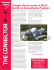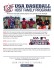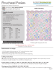Midsegments of Triangles

Transcription

Midsegments of Triangles
LESSON
8.4
Name
Midsegments
of Triangles
Class
8.4
Date
Midsegments of Triangles
Essential Question: How are the segments that join the midpoints of a triangle’s sides related
to the triangle’s sides?
Resource
Locker
Common Core Math Standards
The student is expected to:
COMMON
CORE
The midsegment of a triangle is a line segment that connects the midpoints of two sides of
the triangle. Every triangle has three midsegments. Midsegments are often used_
rigidity to structures. In the support for the garden swing shown, the crossbar DE is a
midsegment of △ABC
Prove theorems about triangles. Also G-CO.D.12, G-GPE.B.4, G-GPE.B.5
Mathematical Practices
COMMON
CORE
Investigating Midsegments of a Triangle
Explore
G-CO.C.10
MP.2 Reasoning
Language Objective
Explain to a partner why a drawn segment in a triangle is or is not a
midsegment.
ENGAGE
Each midsegment is half the length of the side to
which it is parallel; the sum of the lengths of the
midsegments is half the perimeter of the triangle.
PREVIEW: LESSON
View the Engage section online. Discuss the photo,
drawing attention to the horizontal crossbeams that
extend between the slanting beams and stabilize
them. Then preview the Lesson Performance Task.
A
B
Sketch a scalene triangle and label
the vertices A, B, and C.
Use a compass to find the midpoint
¯. Label the midpoint D.
of AB
A
A
D
© Houghton Mifflin Harcourt Publishing Company
Essential Question: How are the
segments that join the midpoints of a
triangle’s sides related to the triangle’s
sides?
You can use a compass and straightedge to construct the midsegments of a triangle.
B
C
B
C
¯. Label
Use a compass to find the midpoint of AC
the midpoint E.
C
_ _
Use a straightedge to draw DE. DE is one of the
midsegments of the triangle.
D
A
A
D
B
E
D
E
E
B
C
C
Repeat the process to _
find the other two midsegments of △ABC. You may want to
label the midpoint of BC as F.
Module 8
be
ges must
EDIT--Chan
DO NOT Key=NL-A;CA-A
Correction
Lesson 4
395
gh “File info”
Date
Class
gles
nts of Trian
Name
Midsegme
d
sides relate
triangle’s
ints of a
the midpo
nts that join
are the segme
ion: How
le’s sides?
.B.5
to the triang
.B.4, G-GPE
.12, G-GPE
es. Also G-CO.D
ms
Triangle
10 Prove theore
8.4
Resource
Locker
Quest
Essential
GE_MNLESE385795_U2M08L4.indd 395
Midseg
stigating
sides of
ints of two
Inve
cts the midpo used to add
_
often
Explore
nt that conne
gments are
ar DE is a
is a line segme
gments. Midse shown, the crossb
a triangle
gment of
three midse
The midse
garden swing
triangle has
rt for the
le. Every
the triang
In the suppo
structures.
rigidity to
of △ABC
gment
midse
htedge to
and straig
a compass
and label
e triangle
Sketch a scalen B, and C.
s A,
the vertice
You can use


A
D
y
g Compan
Publishin
Harcour t
n Mifflin

the
ss to find
Use a compa
int E.
the midpo
A
E
D
C
B

Lesson 8.4
8L4.indd
95_U2M0
ESE3857
midpoint
¯. Label
of AC
find the other
process to _ F.
Repeat the
of BC as
midpoint
label the
Module 8
GE_MNL
C
B
C
B
395
Turn to these pages to
find this lesson in the
hardcover student
edition.
a triangle.
gments of
the midse
construct
int
the midpo
ss to find
D.
Use a compa
midpoint
¯. Label the
of AB
A
395
HARDCOVER PAGES 339346
a
ments of
G-CO.C.
COMMON
CORE
two midse

_ _ is one of the
DE. DE
tedge to draw
le.
Use a straigh
s of the triang
midsegment
A
E
D
C
B
gments of
△ABC. You
may want
to
Lesson 4
395
02/04/14
2:31 AM
02/04/14 2:30 AM
Reflect
1.
2.
3.
EXPLORE
_
_
Use a ruler
the length of DE to the length of BC. What does this tell you
_
_ to compare
?
_
The length of DE is half the length of BC.
Investigating Midsegments
of a Triangle
_
Use a_
protractor to compare m∠ADE and m∠ABC. What does this tell you about DE
and BC? Explain.
_ _
m∠ADE = m∠ABC, so DE ∥ BC since corresponding angles are congruent.
INTEGRATE TECHNOLOGY
a triangle.
A midsegment of a triangle is parallel to the third side of the triangle and is half as long as
Students have the option of completing the
midsegments activity either in the book or online.
the third side.
Explain 1
Describing Midsegments on a
Coordinate Grid
QUESTIONING STRATEGIES
How are the length of the midsegment and the
third side of the triangle related? The
midsegment is one-half the length of the third side.
You can confirm your conjecture about midsegments using the formulas for the midpoint,
slope, and distance.
Example 1

Show that the given midsegment of the triangle is parallel to the third side
of the triangle and is half as long as the third side.
The vertices of △_
GHI are G(-7, -1), H(-5, 5), and I(1, 3). J is
_
_
the
_ midpoint 1of GH, and K_is the midpoint of IH. Show that JK ∥
__
GI and JK = 2 GI. Sketch JK.
(
K 4
)
y1 + y2
x1 + x2 _
,
, to find the
Step 1 Use the midpoint formula, _
2
2
coordinates of J and K.
)
(
EXPLAIN 1
I
x
-8
G
)
-4
-2
0
2
-2
-4
)
_
_
y2 - y1
Step 2 Use _
x 2 - x 1 to compare the slopes of JK and GI.
_ 3 - (-1)
_
1
1 Slope of GI
4-2
Slope of JK = _
=_
=_=_
2
2
1 - (-7)
-2 - (-6)
_ _
Since the slopes are the same, JK ∥ GI.
____
_
_
Step 3 Use √(x 2 - x 1) 2 + (y 2 - y 1) 2 to compare the lengths of JK and GI.
____
JK = √( -2 - (-6) ) 2 + (4 - 2) 2 =
GI =
_
√ 20
Describing Midsegments on a
Coordinate Grid
J
_
-5 + 1 5 + 3
The midpoint of IH is _, _ = (-2, 4). Graph
2
2
_
and label this point K. Use a straightedge to draw JK.
(
y
© Houghton Mifflin Harcourt Publishing Company
(
_
-1 + 5
-7 - 5 , _
= (-6, 2).
The midpoint of GH is _
2
2
Graph and label this point J.
6
H
INTEGRATE MATHEMATICAL
PRACTICES
Focus on Math Connections
MP.1 Remind students that two lines with equal
slopes are parallel and that the slope of a segment is
the difference of its y-coordinates divided by the
difference of its x-coordinates. Also remind them to
subtract the coordinates in the same order.
_
= 2√ 5
―――――――――― = √_
_
80 = 4√ 5
√(1 - (-7)) + (3 - (-1))
2
_
2
_
1 (4√5 ), JK = _
1 GI.
Since 2√5 = _
2
2
Module 8
396
Lesson 4
PROFESSIONAL DEVELOPMENT
GE_MNLESE385795_U2M08L4.indd 396
3/27/14 10:21 AM
Math Background
A midsegment of a triangle is a segment that joins the midpoints of two sides of
the triangle. Together, the three midsegments of a triangle form the sides of the
midsegment triangle. Using the Triangle Midsegment Theorem and the SSS
Triangle Congruence Theorem, it can be proven that the four small triangles
formed by the midsegments are congruent. Since the four triangles together form
the original triangle, each small triangle has one-fourth of its area.
Midsegments of Triangles 396
B
QUESTIONING STRATEGIES
The vertices of △LMN are L(2, 7), M(10, 9), and N(8, 1). P is the
_
_
midpoint of LM, and Q is the midpoint of MN.
_ _
_
Show that PQ ∥ LN and PQ = 1 LN. Sketch PQ.
2
_
To which side of the triangle does the
midsegment appear to be parallel? the side
that does not contain the endpoints of the
midsegment
_ 2 + 10 7 + 9
Step 1 The midpoint of LM = _, _ =
2
2
Graph and label this point P.
(
(6
, 8
)
=
(9
,
)
M
P
8
L
6
)
Q
. 4
2
10
1
8
9
_
+
+
The midpoint of NM = __, __
2
2
How do you find the midsegments of a
triangle in the coordinate plane? Use the
Midpoint Formula to find the coordinates of the
midpoint of two sides of the triangle. Plot the
midpoints and connect them to form a midsegment.
y
10
0
2
4
N
8
6
x
10
_
. Graph and label this point Q. Use a straightedge to draw PQ .
5
_
5-8
Step 2 Slope of PQ = _
= -1
9- 6
1 - 7
_
Slope of LN = __ = -1
8 - 2
_
_
Since the slopes are the same, PQ and LN are parallel .
What are some ways you can show that two
line segments are parallel when using the
Triangle Midsegment Theorem in a coordinate
plane? Sample answer: Show that the lines have the
same slope.
____
Step 3 PQ =
√(
)
2
__
9 - 6 + (5 - 8) =
2
√
_____
LN =
√( 8
- 2
_
Since 3
√2
) +(
2
1 - 7
( √ 2 ),
1
=_
2 6
_
_
___
) = √ 2
_
9 + 9 = √18 = 3√2
36 + 36
_
=
√ _
=6
72
√2
―
1 ―
PQ = _
2 LN .
_
_
The length of PQ is half the length of LN.
© Houghton Mifflin Harcourt Publishing Company
4.
The vertices _
of △XYZ are X(3, 7), Y(9, 11),_
and Z(7, 1). U is the
midpoint
is the midpoint of XZ. Show
_ _of XY, and W_
_ that
1
UW ∥ YZ and UW = YZ. Sketch △XYZ and UW.
2
3 + 9 7 + 11
U = _, _ = (6, 9);
2
2
7+1
3+7 _
_
,
W=
2
2
(
(
= (5, 4);
)
)
― 4-9
slope of UW = _ = 5;
5-6
― 1 - 11 = 5;
slope of YZ = _
― ―
Since the slopes are the same, UW ∥ YZ.
7-9
――――――― ―
―――――――
26 ;
YZ = √(7 - 9) + (1 - 11) = 2 √―
y
Y
10
U
8
6
X
4
W
2
0
x
Z
2
4
6
8
10
UW = √(5 - 6) + (4 - 9) = √26 ;
2
2
2
2
1 YZ.
S0 UW = _
2
Module 8
397
Lesson 4
COLLABORATIVE LEARNING
GE_MNLESE385795_U2M08L4.indd 397
Small Group Activity
Have one student in each group use geometry software to construct the
midsegment of a triangle. Ask another student in the group to measure the
midsegment and the sides of the triangle and make a conjecture about the
relationship of the two measures. Ask a third student to measure the angles to
make a conjecture about midsegment and the third side of the triangle. Have the
fourth student drag the vertices of the triangle to verify the conjectures made by
the other students: the midsegments are half the measure of the third side and are
parallel to the third side.
397
Lesson 8.4
5/22/14 10:14 PM
Explain 2
Using the Triangle Midsegment
Theorem
EXPLAIN 2
The relationship you have been exploring is true for the three midsegments of every triangle.
Using the Triangle
Midsegment Theorem
Triangle Midsegment Theorem
The segment joining the midpoints of two sides of a triangle is parallel to the third
side, and its length is half the length of that side.
INTEGRATE MATHEMATICAL
PRACTICES
Focus on Math Connections
MP.1 Point out that if the midsegments of a triangle
You explored this theorem in Example 1 and will be proving it later in this course.
Example 2

Use triangle RST.
S
Find UW.
are found, then the Triangle Midsegment Theorem
can be used to state that the midsegment is half the
length of the third side and is parallel to the third
side. Explain that the theorem gives a way to
indirectly find the side lengths of a triangle, and
that it gives similar triangles, which will be studied
in Unit 4.
By the Triangle
Midsegment Theorem,_the length of
_
midsegment UW is half the length of ST.
1 ST
UW = _
2
1 (10.2)
UW = _
2
UW = 5.1
U
41°
5.6
V
10.2
R
W

T
Complete the reasoning to find m ∠SVU.
△Midsegment Thm.
_ _
UWǁST
Alt. Int. Angles Thm.
m ∠SVU = m ∠VUW
Substitute
41° for m∠VUW
QUESTIONING STRATEGIES
m ∠SVU = 41°
In the example, how do you know that you can
use the Triangle Midsegment Theorem? The
midsegment of a triangle was found, so the Triangle
Midsegment Theorem applies.
Reflect
How do you know to which side of a triangle a midsegment is parallel?
Since a midsegment connects the midpoints of two sides, it is parallel to the third side.
6.
Find JL, PM, and m ∠MLK.
1 KL = _
1 (95) = 47.5;
JL = 2 (PN) = 2(39) = 78; PM = _
2
2
m ∠MLK = m ∠JMP = 105°
P
J
K
39
105° M
N
95
L
Elaborate
7.
¯ is NOT a midsegment of the triangle.
Discussion Explain why XY
¯ are not midpoints of the sides of
The endpoints of XY
6
5
X
© Houghton Mifflin Harcourt Publishing Company
5.
AVOID COMMON ERRORS
Sometimes students write the incorrect algebraic
expressions to indirectly find the lengths of segments
in triangles when using the Triangle Midsegment
Theorem. Have these students use the theorem to
write the relationships among the segments before
they substitute algebraic expressions for the
segments. The correct expressions should give the
correct equations to solve for the lengths.
6
Y
5
the triangle.
Module 8
398
Lesson 4
DIFFERENTIATE INSTRUCTION
GE_MNLESE385795_U2M08L4 398
Modeling
6/10/15 12:22 AM
Have students cut a large scalene triangle from heavy paper. Have them construct
the midpoints of two sides and connect them, forming a midsegment. Then have
them cut along this segment to form a triangle and a trapezoid. Have them rotate
the small triangle and place it next to the trapezoid to form a parallelogram. Ask
students to make conjectures about the relationship between the midsegment and
the base of the triangle.
Midsegments of Triangles 398
8.
ELABORATE
Essential Question Check–In Explain how the perimeter of △DEF
compares to that of △ABC.
A
QUESTIONING STRATEGIES
F
How could you use a property of midsegments
to find a measurement for something that is
difficult to measure directly? Sample answer: If you
cannot measure directly across something like a
pond, you can construct a triangle around it so that
the distance you want to measure is a midsegment
of the triangle. Then you can measure the third side
of the triangle and divide it by 2 to find the measure
you are looking for.
D
C
E
B
1 perimeter of △ABC .
The perimeter of △DEF = _
)
(
2
Evaluate: Homework and Practice
1.
Use a compass and a ruler or geometry software to construct an obtuse triangle.
Label the vertices. Choose two sides and construct the midpoint of each side; then
label and draw the midsegment. Describe the relationship between the length of the
midsegment and the length of the third side.
• Online Homework
• Hints and Help
• Extra Practice
Drawings will vary. Students should conclude that the midsegment is half
the length of the third side.
SUMMARIZE THE LESSON
2.
Have students fill out a chart to summarize
midsegments. Sample:
_
3.
© Houghton Mifflin Harcourt Publishing Company
Triangle Midsegment
A line segment that
Definition
connects the
midpoints of two
sides of the triangle
Half the length of the
Properties
third side; parallel to
the third side
Example
)
The vertices of △WXY are W(-4, 1), X( 0, -5
Y 4, -1). A is the midpoint of
_, and
_
_
_(
1 WX.
WY, and B is the midpoint of XY. Show that AB ǁWX and AB = _
2
_
_
The coordinates of A and B are (0, 0) and (2, -3). The slope of AB and WX
_
is - 3 , so the lines are parallel. The length of AB is 13 and the length of
2
_
1 WX.
WX is 213 , so AB = _
2
―
―
), and H(-5, –2). X is the midpoint of
The
_ vertices of △FGH are F(-1,
_
_ 1), G( -5, 4_
FG, and Y is the midpoint of FH. Show that XY ǁGH and XY = __12 GH.
5
1 . The slope of _
XY
The coordinates of X and Y are -3, _ and -3, -_
2
2
_
_
and GH is undefined, so the lines are parallel. The length of XY is 3 and
_
1 GH.
the length of GH is 6, so XY = _
2
_
One of the vertices of △PQR is P(3, –2). The midpoint of PQ is M(4, 0).
_
_ _
1 PR.
The midpoint of QR is N(7, 1). Show that MN ǁ PR and MN = _
2
-2
+
y
3+x
_
_ = 0, So y = 2.
= 4, So x = 5;
2
2
The coordinates of Q are (5, 2).
2+y
5+x
_
_
= 7, So x = 9;
= 1, So y = 0.
2
2
The coordinates of R are (9, 0).
1 , so the lines are parallel. The length of ¯
The slope of ¯
MN and ¯
PR is _
MN is
3
_
1
_
¯
√ 10 and the length of PR is 2 √10 , so MN = PR.
2
(
4.
) (
)
―
Module 8
399
Lesson 4
LANGUAGE SUPPORT
GE_MNLESE385795_U2M08L4.indd 399
Communicate Math
Divide students into pairs. Provide each pair with pictures of triangles with
segments joining two sides. Have one student explain why a drawing does or does
not show a midsegment. Encourage the use of the words parallel and midpoint.
Have students measure the distance between the segment and the base at different
intervals to see if the segment and the base are parallel, and to measure the sides
joined by the segment, to see if the segment’s endpoints are the midpoints of the
sides. Students switch roles and repeat the process.
399
Lesson 8.4
3/22/14 2:36 PM
5.
_
3 , 2 . The midpoint
One
(0, 0). The midpoint of AC is J _
_of the vertices of △ABC_is A_
2
1
_
of BC is K (4, 2). Show that JK ǁBA and JK = BA.
2
0+y
0+x _
3
_
=
=2
So, the coordinates of C are (3, 4).
2
2
2
x=3
y=4
( )
_
EVALUATE
Use the midpoint formula to find the coordinates of B (x, y).
4+y
3+x
_
_
=2
=4
2
2
3+x=8
4+y=4
x=5
y=0
ASSIGNMENT GUIDE
So, the coordinates of B are (5, 0).
5
The slope of ¯
JK and ¯
BA is 0, so the lines are parallel. The length of ¯
JK is _
2
_
1 BA.
and the length of BA is 5, so JK = _
2
Find each measure.
A
X
B
6.
8.
68°
Y
4.6
C
Z
15.8
XY
1 BC = _
1 (15.8) = 7.9
XY = _
2
2
7.
9.
m∠YZC
∠YZC and ∠XYZ are alternate interior
angles, so they are congruent.
m∠YZC = 68°
10. m∠BXY
m∠BXY + m∠ZYX = 180° (same-side interior angles)
m∠BXY + 68° = 180°
m∠BXY = 112°
Module 8
Exercise
GE_MNLESE385795_U2M08L4 400
Explore
Investigating Midsegments of a
Triangle
Exercises 1–3
Example 1
Describing Midsegments on a
Coordinate Grid
Exercises 4–5
Example 2
Using the Triangle Midsegment
Theorem
Exercises 6–15
need to do the exercises. Define midsegment. Make a
sketch on the board showing students how to draw a
midsegment, and point out that every triangle has
three midsegments. Add that, in this lesson, students
will primarily work with one midsegment at a time.
Lesson 4
400
Depth of Knowledge (D.O.K.)
Practice
INTEGRATE MATHEMATICAL
PRACTICES
Focus on Math Connections
MP.1 Remind students of the key concepts they
© Houghton Mifflin Harcourt Publishing Company
AX
1 AB, find AB first.
Since AX = _
2
AB = 2(YZ) = 2(4.6) = 9.2
1 AB = _
1 (9.2) = 4.6
AX = _
2
2
BZ
1 BC = _
1 (15.8) = 7.9
BZ = _
2
2
Concepts and Skills
COMMON
CORE
Mathematical Practices
1–15
2 Skills/Concepts
MP.6 Precision
16–21
2 Skills/Concepts
MP.2 Reasoning
22
3 Strategic Thinking
MP.4 Modeling
23
3 Strategic Thinking
MP.6 Precision
24
3 Strategic Thinking
MP.4 Modeling
10/14/14 9:19 PM
Midsegments of Triangles 400
Algebra Find the value of n in each triangle.
INTEGRATE MATHEMATICAL
PRACTICES
Focus on Communication
MP.3 Discuss each of the following statements as a
11.
12.
6n
11.3
48
n + 4.2
class. Have students explain how the Triangle
Midsegment Theorem justifies their responses.
2(11.3) = n + 4.2
1 (48)
6n = _
2
6n = 24
1. A triangle has three midsegments.
22.6 = n + 4.2
18.4 = n
n=4
2. The midsegments form a triangle with a perimeter
equal to one-half the perimeter of the original
triangle.
13.
14.
14n
n + 12
4n + 9
6n
3. The midsegments form a triangle with area equal
to one-fourth the area of the original triangle.
6n = 2(n + 12)
14n = 2(4n + 9)
6n = 2n + 24
14n = 8n + 18
4n = 24
6n = 18
n=6
n=3
15. Line segment XY is a midsegment of △MNP. Determine whether each of the
following statements is true or false. Select the correct answer for each lettered part.
N
© Houghton Mifflin Harcourt Publishing Company
X
M
Y
P
a. MP = 2XY
1 XY
b. MP = _
2
c. MX = XN
1 NX
d. MX = _
2
e. NX = YN
1 MP
f. XY = _
2
True
False
True
False
True
False
True
False
True
False
True
False
16. What do you know about two of the midsegments in an isosceles triangle? Explain.
1
Two of the midsegments will be congruent because their lengths are __
the length of
2
congruent sides.
Module 8
GE_MNLESE385795_U2M08L4.indd 401
401
Lesson 8.4
401
Lesson 4
3/22/14 2:35 PM
17. Suppose you know that the midsegments of a triangle are all 2 units long. What kind
of triangle is it?
PEERTOPEER DISCUSSION
an equilateral triangle with sides 4 units long
Ask students to discuss with a partner how to locate
the midsegments of a triangle drawn in the
coordinate plane. Have them use the slope formula or
distance formula and the Triangle Midsegment
Theorem to verify that the midsegments are equal to
one-half the third side and are parallel to the third
side. Have them draw examples to justify
their reasoning.
_ _
_
18. In △ABC, m∠A = 80°, m∠B = 60°, m∠C = 40°. The midpoints of AB, BC, and AC
are D, E, and F, respectively. Which midsegment will be the longest? Explain how you
know.
¯ is the longest side because it is opposite the largest angle, so midsegment ¯
BC
DF will be
the longest.
19. Draw Conclusions Carl’s Construction is building a pavilion
with an A-frame roof at the local park. Carl has constructed two
triangular frames for the front and back of the roof, similar to
△ABC in the diagram. The base of each frame, represented by
_
AC, is 36 feet _
long. He_
needs to insert a crossbar connecting the
midpoints of AB and BC, for each frame. He has 32 feet of timber
left after constructing the front and back triangles. Is this enough
to construct the crossbar for both the front and back frame?
Explain.
AVOID COMMON ERRORS
When using triangles in the coordinate plane, some
students may assume that the Triangle Midsegment
Theorem applies only to acute, scalene triangles.
Have students work in groups to draw other types of
triangles in the coordinate plane and use algebraic
expressions to write the triangle relationships that
apply to these triangles.
B
D
E
A
C
needs 2 × 18, or 36, feet of timber. He needs 4 more feet.
AB is a midsegment in △PQR.
20. Critique Reasoning Line segment
_
Kayla calculated the length of AB. Her work is shown below. Is her
answer correct? If not, explain her error.
2(QR) = AB
2(25) = AB
50 = AB
R
B
25
Q
A
P
1
No, it is not correct. The equation should have __
QR on the left instead of 2(QR).
2
21. Using words or diagrams, tell how to construct a midsegment using only a
straightedge and a compass.
Possible answer: First construct a triangle with a straightedge. Next use the compass to
find the midpoint of two sides of the triangle. Finally, connect the two midpoints to create
a midsegment parallel to the third side.
Module 8
GE_MNLESE385795_U2M08L4.indd 402
402
Igor/Shutterstock
_
_
No. If AC is 36 feet long, DE is 18 feet long. Because he needs to insert two crossbars, he
Lesson 4
5/22/14 10:16 PM
Midsegments of Triangles 402
JOURNAL
H.O.T. Focus on Higher Order Thinking
Have students explain how to find the length of a
midsegment when given the length of the
parallel side.
22. Multi–Step A city park will be shaped like a right triangle,
_
and _
there will be two pathways for pedestrians, shown by VT
and VW in the diagram. The park planner only wrote two
lengths on his sketch as shown. Based on the diagram, what
will be the lengths of the two pathways?
Y
30 yd
X
V
W
Z
T
24 yd
VW =
_1 (XZ) = _1 (24) = 12
2
2
2
24 2 + (YZ) = 30 2 (Pyth. Thm.)
VT =
_1 (YZ) = _1 (18) = 9
2
2
VW = 12 yd; VT = 9 yd
576 + (YZ) = 900
2
(YZ) 2 = 324
YZ = 18
Hope/iStockPhoto.com
23. Communicate Mathematical Ideas △XYZ is the midsegment of △PQR.
Write a congruence statement involving all four of the smaller triangles. What
is the relationship between the area of △XYZ and △PQR?
Q
△QXY ≅ △XPZ ≅ △YZR ≅ △ZYX;
area of △XYZ =
Y
X
P
__1 area of △PQR
4
_
_
24. Copy the diagram shown. AB is a midsegment of △XYZ. CD is a
midsegment of △ABZ.
AB? What is the ratio of AB to XY?
a. What is the length of ¯
1
AB = (XY), so AB is 32. The ratio of AB to XY is 32:64, or 1:2.
2
CD? What is the ratio of CD to XY?
b. What is the length of ¯
1
CD = (AB), so CD is 16. The ratio of CD to XY is 16:64, or 1:4.
2
_
c. Draw EF such that points E and F are __34 the distance from point Z
to _
points X and Y. What is the ratio of EF to XY? What is the length
of EF?
3
3
EF = (XY) = (64) = 48
4
4
The length of ¯
EF is 48, so the ratio of EF to XY is 48:64, or 3:4.
Z
C
_
D
A
B
E
_
_
R
Z
X
F
64
Y
_
d. Make a conjecture about the length of non-midsegments when compared to the
length of the third side.
The length of the non-midsegment compared to the length of the third side is the same as
the ratio of the distance of the segment from the vertex opposite the third side compared
to the whole triangle.
Module 8
GE_MNLESE385795_U2M08L4.indd 403
403
Lesson 8.4
403
Lesson 4
5/22/14 10:21 PM
AVOID COMMON ERRORS
The figure shows part of a common roof design
using
stable triangular trusses.
_ and_
_ _
_very
_strong
Points B, C, D, F, G, and I are midpoints of AC, AE, CE, GE, HE and AH respectively. What is
the total length of all the stabilizing bars inside △AEH? Explain how you found the answer.
Students may mistakenly assume that because B, C,
_
and D divide AE into four congruent segments, G
_
and F must divide HE into three congruent segments,
_
and that J and I divide AH into three congruent
segments. Point out that because G is the midpoint of
_
HE, GF and FE are each only half of HG. Similarly,
_
because I is the midpoint of AH, AJ and JI are each
only half of IH.
A
J
20 ft
B
C
I
4 ft
D
H
G
F
14 ft
E
INTEGRATE MATHEMATICAL
PRACTICES
Focus on Critical Thinking
MP.3 On the roof truss diagrammed below,
D, E,
_ _
By the Midsegment Theorem, IC = 7 ft and CG = 10 ft because they are, respectively, half
of HE and AH. CH = 8 ft because it is twice DG. DF = 5 ft, JB = 3.5 ft, and IB = 4 ft because
they are, respectively, half of CG, IC, and HC.
Total length: JB + IB + IC + CH + CG + DG + DF = 3.5 + 4 + 7 + 8 + 10 + 4 + 5 = 41.5 ft
and F are the midpoints of, respectively, AC, AB,
_
and CB.
A
E
D
© Houghton Mifflin Harcourt Publishing Company
Module 8
404
C
F
B
Compare the perimeter and area of ▵ABC with those
Students can use the Midsegment Theorem and the
_ _
_
facts that DE, EF, and DF are parallel to and half the
_ _
_
length of, respectively, CB, AC, and AB to establish
that (1) the perimeter of ▵DEF is half that of ▵ABC,
and (2) the four small triangles in the diagram are
congruent, which means that the area of ▵DEF is
one-fourth that of ▵ABC.
Lesson 4
EXTENSION ACTIVITY
GE_MNLESE385795_U2M08L4.indd 404
Have students research tri-bearing roof trusses. Among topics they can report on:
3/22/14 2:35 PM
• the definition of tri-bearing roof truss;
• the design of such a truss, together with a drawing and typical measurements;
• the meaning of the term tri-bearing;
• a description of the relationship between tri-bearing roof trusses and the
Midsegment Theorem.
Scoring Rubric
2 points: Student correctly solves the problem and explains his/her reasoning.
1 point: Student shows good understanding of the problem but does not fully
solve or explain.
0 points: Student does not demonstrate understanding of the problem.
Midsegments of Triangles 404

5.1 Midsegment Theorem and Coordinate Proof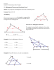Geometry     Chapter  5:  Relationships  within  Triangles

wizBRAIN - W4Kangoeroe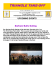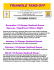Binomial`s power There is a formula which links the power of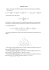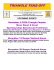Simpletones: A System of Collaborative Physical Controllers for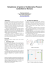Triangle Electric works at NASA facility on Constellation Program